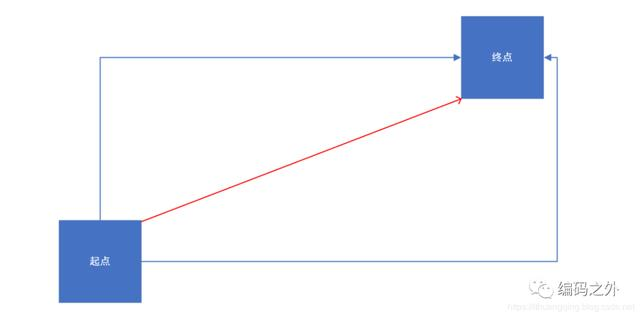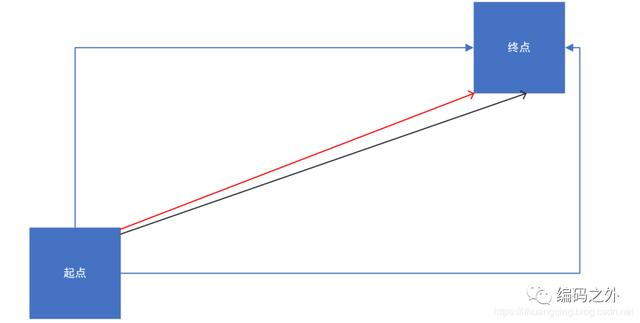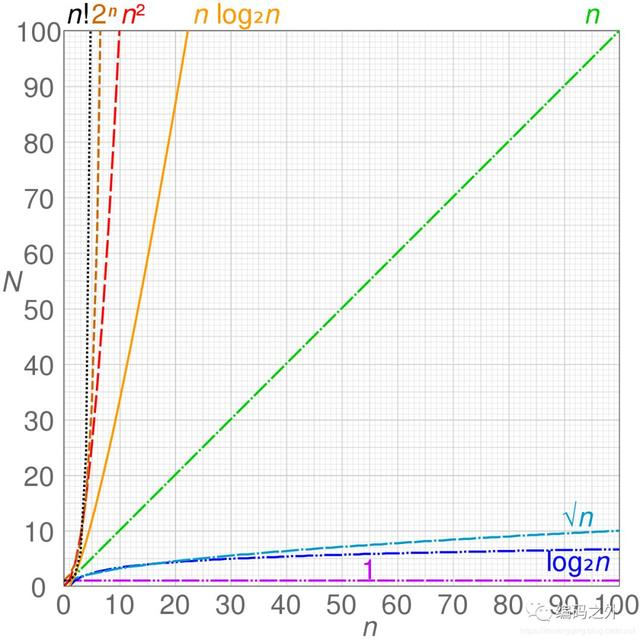public static int test2(int n) {if(n<=1) return n;int first = 0;int second = 1;for (int i = 1; i < n; i++) {second += first;first = second - first;}return second;}

public static int test(int n) {return n++;}

return n++;

public static int test(int n) {n=+5;return n++;}

public static int test(int n) {int sum = 0;for (int i=0;i<n;i++) {sum=+i;}return sum;}